RD Sharma 2013 Solutions for Class 6 Math Chapter 20 Mensuration are provided here with simple step-by-step explanations. These solutions for Mensuration are extremely popular among class 6 students for Math Mensuration Solutions come handy for quickly completing your homework and preparing for exams. All questions and answers from the RD Sharma 2013 Book of class 6 Math Chapter 20 are provided here for you for free. You will also love the ad-free experience on Meritnation’s RD Sharma 2013 Solutions. All RD Sharma 2013 Solutions for class 6 Math are prepared by experts and are 100% accurate.

#### Question 1:

Find the perimeters of the rectangles whose lengths and breadths are given below:

(i) 7 cm, 5 cm
(ii) 5 cm, 4 cm
(iii) 7.5 cm, 4.5 cm

#### Answer:

(i) Perimeter of a rectangle  =  2$×$(Length + Breadth)
$\because$ Length = 7 cm, Breadth = 5 cm
$\therefore$ Perimeter = 2 $×$(7 + 5) = 2 $×$ (12) = 24 cm

(ii) Perimeter of a rectangle  =  2 $×$ (Length + Breadth)
$\because$ Length = 5 cm, Breadth = 4 cm
$\therefore$ ​Perimeter = 2 $×$ (5 + 4) = 2 $×$ (9) = 18 cm

(iii) Perimeter of a rectangle  =  2 $×$ (Length + Breadth)
$\because$ Length = 7.5 cm, Breadth = 4.5 cm
$\therefore$ P​erimeter = 2 $×$ (7.5 + 4.5) = 2 $×$ (12) = 24 cm

#### Question 2:

Find the perimeters of the squares whose sides are given below:

(i) 10 cm
(ii) 5 m
(iii) 115.5 cm

#### Answer:

Perimeter of a square = 4 $×$ (Length of one side)

(i) Length of one side = 10 cm
Perimeter = 4 $×$ 10 = 40 cm

(ii) Length of one side = 5 m
Perimeter = 4 $×$ 5 = 20 m

(iii) Length of one side = 115.5 cm
Perimeter = 4 $×$ 115.5 = 462 cm

#### Question 3:

Find the side of the square whose perimeter is:

(i) 16 m
(ii) 40 cm
(iii) 22 cm

#### Answer:

Side of a square =
(i) Perimeter = 16 m
$\therefore$ Side of this square =  = 4 m
(ii) Perimeter = 40 cm
$\therefore$ Side of this square = $\frac{40}{4}$ = 10 cm
(iii) Perimeter = 22 cm
$\therefore$ Side of this square = $\frac{22}{4}$ = 5. 5cm

#### Question 4:

Find the breadth of the rectangle whose perimeter is 360 cm and whose length is

(i) 116 cm
(ii) 140 cm
(iii) 102 cm

#### Answer:

Perimeter of a rectangle = 2 (Length + Breadth)

$\therefore$ Breadth of the rectangle = $\frac{\mathrm{Perimeter}}{2}-$ Length

(i)
Perimeter = 360 cm
Length = 116 cm

$\therefore$ Breadth = $\frac{360}{2}-116$ = 180 $-$116 = 64 cm

(ii)
Perimeter = 360 cm
Length = 140 cm

$\therefore$ Breadth = $\frac{360}{2}-140$ = 180 $-$140 = 40 cm
(iii)
Perimeter = 360 cm
Length = 102 cm

Breadth = $\frac{360}{2}-102$ = 180 $-$ 102 = 78 cm

#### Question 5:

A rectangular piece of lawn is 55 m wide and 98 m long. Find the length of the fence around it.

#### Answer:

Length of the lawn = 98 m
Breadth of the lawn = 55 m
Length of the fence around the lawn = Perimeter of the lawn = 2 $×$ (Length + Breadth)
Perimeter of the lawn = 2 $×$ (98 + 55) m = 2 $×$(153) = 306 m
Thus, the length of the fence around the lawn = 306 m

#### Question 6:

The side of a square field is 65 m. What is the length of the fence required all around it?

#### Answer:

Side of the square field = 65 m
Length of the fence around the square field = Perimeter of the square field = 4 $×$ (Side of the square)
Perimeter of the square field = 4 $×$ 65 = 260 m
Thus, the length of the fence around the square filed = 260 m

#### Question 7:

Two sides of a triangle are 15 cm and 20 cm. The perimeter of the triangle is 50 cm. What is the third side?

#### Answer:

Given:
Perimeter = 50 cm
Length of the first side = 15 cm
Length of the second side = 20 cm
We have to find the length of the third side.
Perimeter of a triangle = Sum of all three sides of the triangle
$\therefore$ Length of the third side = (Perimeter of the triangle) $-$ (Sum of the length of the other two sides)
= 50 $-$ (15 + 20)
= 50 $-$ 35 = 15 cm

#### Question 8:

A wire of length 20 m is to be folded in the form of a rectangle. How many rectangles can be formed by folding the wire if the sides are positive integers in metres ?

#### Answer:

It is given that a wire of length 20 m is to be folded in the form of a rectangle; therefore, we have:
Perimeter  of the rectangle = 20 m

$⇒$ 2(Length + Breadth) = 20 m
$⇒$ (Length + Breadth ) = 20/2 = 10 m

Since, length and breadth are positive integers in metres, therefore, the possible dimensions are:
(1m, 9m), (2m, 8m), (3m, 7m), (4m, 6m) and (5m, 5m)

Thus, five rectangles can be formed with the given wire.

#### Question 9:

A square piece of land has each side equal to 100 m. If 3 layers of metal wire has to be used to fence it, what is the length of the wire needed?

#### Answer:

Side of the square field = 100 m
Wire required to fence the square field = Perimeter of the square field = 4 $×$ Side of the square field
Perimeter = 4 $×$100 = 400 m
This perimeter is the length of wire required to fence one layer.
Therefore, the length of wire required to fence three layers = 3 $×$ 400 m = 1200 m

#### Question 10:

Shikha runs around a square of side 75 m. Priya runs around a rectangle with length 60 m and breadth 45 m. Who covers the smaller distance?

#### Answer:

Shikha and Priya, while running around the square and rectangular field respectively, actually cover a distance equal to the perimeters of these fields.
$\therefore$ Distance covered by Shikha = Perimeter of the square = 4 $×$ 75 m = 300 m
Similarly, distance covered by Priya = Perimeter of the rectangle = 2 $×$ (60 + 45) = 2 $×$ 105 = 210 m
Thus, it is evident that the distance covered by Priya is less than that covered by Shikha.

#### Question 11:

The dimensions of a photographs are 30 cm × 20 cm. What length of wooden frame is needed to frame the picture?

#### Answer:

Dimensions of the photograph = 30 cm $×$ 20 cm
So, the required length of wooden frame = Perimeter of the photograph                                                                                                                               = 2 (Length + Breadth)
= 2 $×$ (30 + 20) cm
= 2 $×$ 50 cm
= 100 cm

#### Question 12:

The length of a rectangular fields is 100 m. If its perimeter is 300 m, what is its breadth?

#### Answer:

Length of the rectangular field = 100 m
Perimeter of the rectangular field  = 300 m
Perimeter of a rectangle = 2 (Length + Breadth)
Applying the above formula, we get:
Breadth of the rectangular field = $\frac{Perimeter}{2}-Length$ = $\frac{300}{2}-100$ = 150 $-$ 100 = 50 m

#### Question 13:

To fix fence wires in a garden, 70 m long and 50 m wide, Arvind bought metal pipes for bosts, he fixed a post every 5 metres apart. Each post was 2 m long. What is total length of the pipes he bought for the posts?

#### Answer:

Length of the garden = 70 m
Breadth of the garden = 50 m
Perimeter of the garden = 2 $×$(Length + Breadth)
= 2 $×$ (70 + 50)
= 2 $×$ 120 = 240 m
On the perimeter of the garden​, it is given that Arvind fixes a post every 5 metres apart.

So, the number of posts required = $\frac{240}{5}$  = 48
$\because$ Length of each post = 2 m
$\therefore$ Total length of the pipe required = 48 $×$2 = 96 m

#### Question 14:

Find the cost of fencing a rectangular park of length 175 m and breadth 125 m at the rate of Rs 12 per meter.

#### Answer:

Length of the park = 175 m
Breadth of the park = 125 m
Perimeter of the park = 2$×$(Length + Breadth)
= 2 $×$(175 + 125)
= 2 $×$ 300 = 600 m
Rate of fencing = Rs. 12 per meter
Cost of fencing = Rs. 12 $×$ 600 = Rs. 7,200

#### Question 15:

The perimeter of a regular pentagon is 100 cm. How long is each sides?

#### Answer:

A regular pentagon  is a closed polygon having five sides of equal length.
Perimeter of the regular pentagon = 100 cm
Perimeter of the regular pentagon = 5 $×$ Side of the regular pentagon
Therefore, side of the regular pentagon = $\frac{Perimeter}{5}$ = $\frac{100}{5}$ = 20 cm

#### Question 16:

Find the perimeter of a regular hexagon with each side measuring 8 m.

#### Answer:

A regular hexagon is a closed polygon having six sides of equal lengths.
Side of the hexagon = 8 m
Perimeter of the hexagon = 6$×$ Side of the hexagon
= 6 $×$8 = 48 m

#### Question 17:

A rectangular piece of land measure 0.7 km by 0.5 km. Each side is to be fenced with four rows of wires. What length of the wire is needed?

#### Answer:

Dimensions of the rectangular land = 0.7 km $×$ 0.5 km
Perimeter of the rectangular land = 2 (Length + Breadth)
= 2 (0.7 + 0.5) km = 2 $×$1.2 km = 2.4 km
This perimeter is equal to one row of wire required to fence the land.
Therefore, length of wire required to fence the land with four rows of wire = 4 $×$2.4 km
= 9.6 km

#### Question 18:

Avneet buys 9 square paving slabs, each with a side of $1}{2}$ m. He lays them in the form of a square.(i) What is the perimeter of his arrangement?
(ii) Shari does not like his arrangement. She gets him to lay them out like a cross. What is the perimeter of her arrangement?
(iii) Which has greater perimeter?
(iv) Avneet wonders, If there is a way of getting an even greater perimeter. Can you find a way of doing this? (The paving slabs must meet along complete edges they cannot be broken)

#### Answer:

(i) Length of the side of one slab = 1/2 m
In the square arrangement, one side of the square is formed by three slabs.
So, length of the side of the square = 3 $×\frac{1}{2}$= 3/2 m
The perimeter of the square arrangement = 4 $×\frac{3}{2}$ = 6 m
(ii) The cross arrangement consists of 8 sides. These sides form the periphery of the arrangement and measure 1 m each.
Also, this arrangement consists of other 4 sides that measure 1/2 m each.
So, the perimeter of the cross arrangement = (1 + 1/2 + 1 + 1 + 1/2 + 1 + 1 + 1/2 + 1 + 1 + 1/2 + 1)
= (8 + 2) = 10 m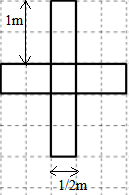(iii) Perimeter of the cross arrangement = 10 m
Perimeter of the square arrangement = 6 m
Thus, the perimeter of the the cross arrangement is more than that of the square arrangement.

(iv) No, there is no way of arranging these slabs where the perimeter is more than 10 m.

#### Question 1:

The following figures are drawn on a squared paper. Count the number of squares enclosed by each figure and find its area, taking the area of each squares as 1 cm2. (Fig.20.25).#### Answer:

(i) There are 16 complete squares in the given shape.
$\because$ Area of one square = 1 cm2
$\therefore$ Area of this shape = 16 $×$ 1 = 16 cm2
(ii) There are 36 complete squares in the given shape.
$\because$ Area of one square = 1 cm2
$\therefore$ Area of 36 squares = 36 $×$1 = 36 cm2
(iii) There are 15 complete and 6 half squares in the given shape.
$\because$ Area of one square = 1 cm2
$\therefore$ Area of this shape = (15 + 6 $×$$\frac{1}{2}$) = 18 cm2
(iv) There are 20 complete and 8 half squares in the given shape.
$\because$ Area of one square = 1 cm2
$\therefore$ Area of this shape = (20 + 8$×$ $\frac{1}{2}$) = 24 cm2
(v) There are 13 complete squares, 8 more than half squares and 7 less than half squares in the given shape.
$\because$ Area of one square = 1 cm2
$\therefore$ Area of this shape = (13 + 8$×$1) = 21 cm2

(vi) There are 8 complete squares, 6 more than half squares and 4 less than half squares in the given shape.
$\because$ Area of one square = 1 cm2
$\therefore$ Area of this shape = (8 + 6 $×$ 1) = 14 cm2

#### Question 2:

On a squared paper, draw (i) a rectangle, (ii) a triangle (iii) any irregular closed figure. Find the approximate area of each by counting the number of squares complete, more than half and exactly half.

#### Answer:

(i)  A rectangle: This contains 18 complete squares.
If we assume that the area of one complete square is 1 cm2, then the area of this rectangle will be 18 cm2.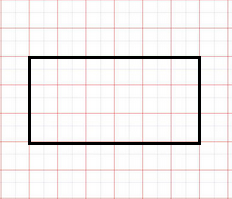(ii) A triangle: This triangle contains 4 complete squares, 6 more than half squares and 6 less than half squares.
If we assume that the area of one complete square is 1 cm2, then the area of this shape = (4 + 6 $×$ 1) = 10 cm2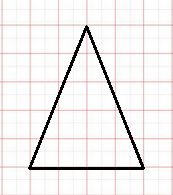(iii) Any irregular figure: This figure consists of 10 complete squares, 1 exactly half square, 7 more than half squares and 6 less than half squares.
If we assume that the area of one complete square is 1 cm2, then the area of this shape = (10 +  + 7 $×$ 1) = 17.5 cm2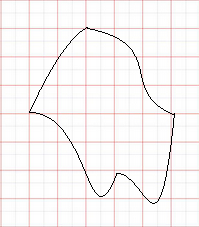#### Question 3:

Draw any circle on the graph paper. Count the squares and use them to estimate the area of the circular region.
Figure

#### Answer: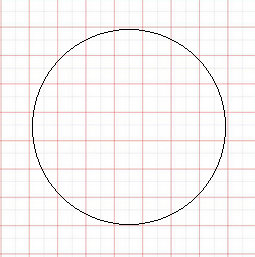This circle on the squared paper consists of 21 complete squares, 15 more than half squares and 8 less than half squares.
Let us assume that the area of 1 square is 1 cm2.
If we neglect the less than half squares while approximating more than half square as equal to a complete square, we get:
Area of this shape = (21 + 15) = 36 cm2

#### Question 4:

Use tracing paper and centimeter graph paper to compare the areas of the following pairs of figures:#### Answer: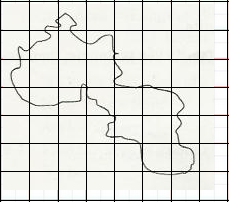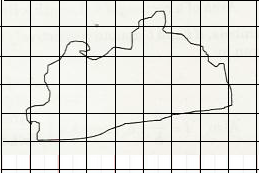(i)                                                                                        (ii)
Using tracing paper, we traced both the figures on a graph paper.
(i) This figure contains 4 complete squares, 9 more than half squares and 9 less than half squares.
Let us assume that the area of one square is 1 cm2 .
If we neglect the less than half squares and consider the area of more than half squares as equal to area of complete square, we get:
Area of this shape = (4 + 9) = 13 cm2
(ii) This figure contains 8 complete squares, 11 more than half squares and 10 less than half squares.
Let us assume that the area of one square is 1 cm2​.
If we neglect the less than half squares and consider the area of more than half squares as equal to area of complete square, we get:
Area of this shape = (8 + 11) = 19 cm2

On comparing the areas of these two shapes, we get that the area of Fig. (ii) is more than that of Fig. (i).

#### Question 1:

Find the area of a rectangle, whose

(i) Length = 6 cm, breadth = 3 cm
(ii) Length = 8 cm, breadth = 3 cm
(iii) Length = 4.5 cm, breadth = 2 cm.

#### Answer:

(i)
Area of a rectangle = Length $×$ Breadth
Length = 6 cm
Breadth = 3 cm
$\therefore$ Area of rectangle = 6 $×$3 = 18 cm2
(ii)
Area of a rectangle = Length $×$ Breadth
Length = 8 cm
Breadth = 3 cm
$\therefore$ Area of rectangle = 8 $×$3 = 24 cm2
(iii)
Area of a rectangle = Length $×$ Breadth
Length = 4.5 cm
Breadth =2 cm
$\therefore$ Area of rectangle = 4.5 $×$2 = 9 cm2

#### Question 2:

Find the area of a square whose side is:

(i) 5 cm
(ii) 4.1 cm
(iii) 5.5 cm
(iv) 2.6 cm

#### Answer:

Area of a square = Side $×$ Side
(i) Side of the square = 5 cm
Area of the square = 5 $×$5 = 25 cm2
(ii) Side of the square = 4.1 cm
Area of the square = 4.1 $×$ 4.1 = 16.81 cm2
(iii) Side of the square = 5.5 cm
Area of the square = 5.5 $×$ 5.5 = 30.25 cm2
(iv) Side of the square = 2.6 cm
Area of the square = 2.6 $×$ 2.6 = 6.76 cm2

#### Question 3:

The area of a rectangle is 49 cm2 and its breadth is 2.8 cm, find the length of the rectangle.

#### Answer:

Area = 49 cm2
Breadth = 2.8 cm
Area of the rectangle = Length $×$ Breadth
$\therefore$ Length = = $\frac{49}{2.8}$ = 17.5 cm

#### Question 4:

The side of a square is 70 cm. Find its area and perimeter.

#### Answer:

Side of the square = 70 cm
Area of the square = Side $×$Side
= 70$×$70 = 4900 cm2
Perimeter of the square = 4 $×$ Side = 4 $×$ 70 = 280 cm

#### Question 5:

The area of a rectangle is 225 cm2 and its one side is 25, find its other side.

#### Answer:

Area = 225 cm2
One of the sides = 25 cm
Area of the rectangle = Product of the lengths of its two sides
Other side = = $\frac{225}{25}$ = 9 cm

#### Question 6:

What will happen to the area of a rectangle if its

(i) Length and breadth are trebled.
(ii) Length is doubled and breadth is same.
(iii) Length is doubled and breadth is halved.

#### Answer:

(i) If the length and breadth of a rectangle are trebled.
Let the initial length and breadth be l and b, respectively.
Original area = l $×$b = lb
Now, the length and breadth are trebled which means they become three times of their original value.
Therefore
New length = 3l
New breadth = 3b
New area  =  3l $×$3b = 9 lb
Thus, the area of the rectangle will become 9 times that of its original area.

(ii) If the length is doubled and the breadth is same.
Let the initial length and breadth be l and b, respectively.​
Original area = l $×$b = lb
Now, length is doubled and breadth remains same.
Therefore
New length = 2l

New breadth = b
New area  =  2l $×$b = 2 lb
Thus, the area of the rectangle will become 2 times that of its original area.

(iii) If the Length is doubled and breadth is halved.
Let the initial length and breadth be l and b, respectively.​
Original area = l $×$b = lb
Now, length is doubled and breadth is halved.
Therefore
New length = 2l

New breadth = b/2
New area  =  2l $×$b/2 = lb
New area is also lb. This means that the areas remain the same.

#### Question 7:

What will happen to the area of a square if its side is

(i) Tripled
(ii) increased by half of it.

#### Answer:

(i) Let the original side of the square be s.
Original area = s $×$ s = s2
If the side of a square is tripled, new side will be equal to 3s.
New area = 3s $×$ 3s = 9s2
This means that the area becomes 9 times that of the original area.
(ii) Let the original side of the square be s.
Original area = s $×$s = s2
If the side of a square is increased by half of it, new side = (s +$\frac{1}{2}$s) = $\frac{3}{2}$s
New area = $\frac{3}{2}$s $×$$\frac{3}{2}$s = $\frac{9}{4}$s
This means that the area becomes $\frac{9}{4}$ times that of the original area.

#### Question 8:

Find the perimeter of a rectangle whose area is 500 cm2 and breadth  is 20 cm.

#### Answer:

Area = 500 cm2
Breadth = 20 cm
Area of rectangle = Length $×$ Breadth
Therefore
Length = = $\frac{500}{20}$ = 25 cm
Perimeter of a rectangle = 2 (Length + Breadth)
= 2 (25 + 20) cm = 2 $×$ 45 cm = 90 cm

#### Question 9:

A rectangle has the area equal to that of a square of side 80 cm. If the breadth of the rectangle is 20 cm, find its length.

#### Answer:

Side of the square = 80 cm
Area of square = Side $×$ Side = 80 $×$ 80 = 6400 cm2
Given that:
Area of the rectangle = Area of the square = 6400 cm2
Breadth of the rectangle = 20 cm
Applying the formula:
Length of the rectangle = $\frac{Area}{Breadth}$
We get:
Length of the rectangle = $\frac{6400}{20}$ = 320 cm

#### Question 10:

Area of a rectangle of breadth 17 cm is 340 cm2. Find the perimeter of the rectangle.

#### Answer:

Area of the rectangle = 340 cm2
Breadth of the rectangle = 17 cm
Applying the formula:
Length of a rectangle = $\frac{Area}{Breadth}$
We get:
Length of the rectangle = $\frac{340}{17}$ =20 cm
Perimeter of  rectangle = 2 (Length + Breadth)
= 2 (20 + 17)
= 2 $×$ 37
= 74 cm

#### Question 11:

A marble tile measures 15 cm × 20 cm. How many tiles will be required to cover a wall of size 4 m × 6 m? Also, find the total cost of the tiles at the rate of Rs 2 per tile.

#### Answer:

Dimensions of the tile = 15 cm × 20 cm
Dimensions of the wall = 4 m × 6 m = 400 cm × 600 cm (Since, 1 m = 100 cm, so, 4 m = 400 cm and 6 m = 600 cm)
Area of the tile = 15 cm × 20 cm = 300 cm2
Area of the wall = 400 cm × 600 cm = 2,40,000 cm2

Number of tiles required to cover the wall = = $\frac{240000}{300}$ = 800 tiles

#### Question 12:

A marble tile measures 10 cm × 12 cm. How many tiles will be required to cover a wall of size
3 m × 4 m? Also, find the total cost of the tiles at the rate of Rs 2 per tile.

#### Answer:

Dimension of the tile = 10 cm × 12 cm
Dimension of the wall =3 m × 4 m = 300 cm × 400 cm (Since, 1 m = 100 cm, so, 3 m = 300 cm and 4 m = 400 cm)
Area of the tile = 10 cm × 12 cm = 120 cm2
Area of the wall = 300 cm × 400 cm =1,20,000 cm2
Number of tiles required to cover the wall =  $\frac{120000}{120}$ = 1,000 tiles
Cost of  tiles at the rate of Rs. 2 per tile = 2 × 1,000 = Rs. 2,000

#### Question 13:

One side of a square plot is 250 m, find the cost of levelling it at the rate of Rs 2 per square metre.

#### Answer:

Side of the square plot = 250 m
Area of the square plot = Side $×$ Side = 250 × 250 = 62,500 m2
Rate of levelling the plot = Rs. 2 per m2
Cost of levelling the square plot = Rs. 62,500 × 2 = Rs. 1,25,000

#### Question 14:

The following figures have been split into rectangles. Find their areas. (The measures are given in centimetres)#### Answer:

(i)  This figure consists of two rectangles II and IV and two squares I and III.
Area of square I =  Side × Side = 3 × 3 = 9 cm2
Similarly, area of rectangle II = (2 × 1)  = 2 cm2
area of square III = (3× 3)  = 9 cm2
area of rectangle IV = (2 × 4)  = 8 cm2
Thus, the total area of this figure = (Area of square I + Area of rectangle II + Area of square III + Area of rectangle IV) = 9 + 2 + 9 + 8 = 28 cm2(ii)  This figure consists of three rectangles I, II and III.
Area of rectangle I =  Length × Breadth = 3 × 1 = 3 cm2
Similarly, area of rectangle II = (3 × 1)  = 3 cm2
area of rectangle III = (3 × 1)  = 3 cm2
Thus, the total area of this figure = (Area of rectangle I + area of rectangle II + area of rectangle III) = 3 + 3 + 3 = 9 cm2#### Question 15:

Split the following shapes into rectangles and find the area of each. (The measures are given in centimetres)#### Answer:

(i)  This figure consists of two rectangles I and II.
The area of rectangle I = Length $×$ Breadth = 10 $×$2 = 20 cm2
Similarly, area of rectangle II = 10 $×$$\frac{3}{2}$ = 15 cm2
Thus, total area of this figure = (Area of rectangle I + Area of rectangle II) = 20 + 15= 35 cm2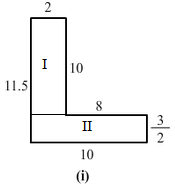(ii)  This figure consists of two squares I and III and one rectangle II.
Area of square I = Area of square III = Side $×$ Side = 7 $×$ 7 = 49 cm2
Similarly, area of rectangle II = (21 $×$ 7)  = 147 cm2
Thus, total area of this figure = (Area of square I + Area of rectangle II + Area of square III) = 49 + 49 + 147 = 245 cm2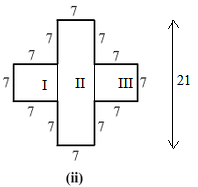(iii) This figure consists of two rectangles I and II.
Area of rectangle I = Length $×$ Breadth = 5 $×$ 1 = 5 cm2
Similarly, area of rectangle II = 4 $×$1 = 4 cm2
Thus, total area of this figure = (Area of rectangle I + Area of rectangle II) = 5 + 4  = 9 cm2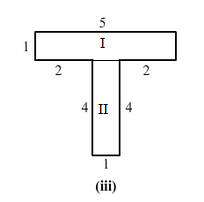#### Question 16:

How many tiles with dimensions 5 cm and 12 cm will be needed to fit a region whose length and breadth are respectively:

(i) 100 cm and 144 cm
(ii) 70 cm and 36 cm

#### Answer:

(i)  Dimension of the tile = 5 cm × 12 cm
Dimension of the region = 100 cm × 144 cm
Area of the tile = 5 cm × 12 cm = 60 cm2
Area of the region = 100 cm × 144 cm = 14,400 cm2
Number of tiles required to cover the region =  $\frac{14400}{60}$ = 240 tiles
(ii)  Dimension of the tile = 5 cm × 12 cm
Dimension of the region = 70 cm × 36 cm
Area of the tile = 5 cm × 12 cm = 60 cm2
Area of the region = 70 cm × 36 cm = 2,520 cm2
Number of tiles required to cover the region =  $\frac{2520}{60}$ = 42 tiles

#### Question 1:

The side of a rectangle are in the ratio 5 : 4. If its perimeter is 72 cm, then its length is

(a) 40 cm
(b) 20 cm
(c) 30 cm
(d) 60 cm

#### Answer:

(b) 20 cm
Let the sides of the rectangle be 5x and 4x. (Since, they are in the ratio 5 : 4)
Now, perimeter of rectangle =  2 (Length + Breadth)
72 = 2 (5x + 4x)
72 = 2 $×$ 9x
72 = 18x
x = $\frac{72}{18}$ = 4
Thus, the length of the rectangle = 5x = 5 $×$ 4 = 20 cm

#### Question 2:

The cost of fencing a rectanglular field 34 m long and 18 m wide at Rs 2.25 per metre is

(a) Rs 243
(b) Rs 234
(c) Rs 240
(d) Rs 334

#### Answer:

(b) Rs. 234
For fencing the rectangular field, we need to find the perimeter of the rectangle.
Length of the rectangle = 34m
Breadth of the rectangle​ = 18m
Perimeter of the rectangle = 2 (Length + Breadth)
= 2 (34 + 18) m
= 2 $×$ 52 m = 104 m
Cost of fencing the field at the rate of Rs. 2.25 per meter = Rs. 104 $×$ 2.25 = Rs. 234

#### Question 3:

If the cost of fencing a rectangular field at Rs. 7.50 per metre is Rs. 600, and the length of the field is 24 m, then the breadth of the field is

(a) 8 m
(b) 18 m
(c) 24 m
(d) 16 m

#### Answer:

(d) 16 m
Cost of fencing the rectangular field = Rs. 600
Rate of fencing the field = Rs. 7.50 per m
Therefore, perimeter of the field = $\frac{600}{7.50}$ = 80 m
Now, length of the field = 24 m
Therefore, breadth of the field = $\frac{Perimeter}{2}-$ Length
= $\frac{80}{2}-$ 24 = 16 ​m

#### Question 4:

The cost of putting a fence around a square field at Rs 2.50 per metre is Rs 200. The length of each side of the field is

(a) 80 m
(b) 40 m
(c) 20 m
(d) None of these

#### Answer:

(c) 20 m

Cost of fencing the square field = Rs. 200

Rate of fencing the field = Rs. 2.50

Now, perimeter of the square field = = $\frac{200}{2.50}$ = 80 m

Perimeter of square = 4 $×$ Side of the square

Therefore, side of the square = $\frac{Perimeter}{4}$ = $\frac{80}{4}$ = 20 m

#### Question 5:

The length of a rectangle is three times of its width. If the length of the diagonal is $8\sqrt{10}$ m, then the perimeter of the rectangle is

(a)
(b)
(c)
(d) 64 m

#### Answer:

(d) 64 m
Let us consider a rectangle ABCD.
Also, let us assume that the width of the rectangle, i.e., BC be x m.
It is given that the length is three times width of the rectangle.
Therefore, length of the rectangle, i.e., AB = 3x m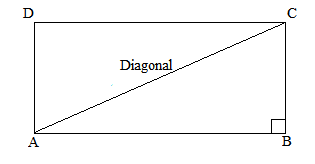Now, AC is the diagonal of rectangle.
In right angled triangle ABC.
AC2  =  AB2  +  BC2                                 {Using Pythagoras theorem}

640 = 9x2  +  x2
640 = 10x2
x2 = $\frac{640}{10}$ =  64
x  = $\sqrt{64}$ = 8 m

Thus, breadth of the rectangle  = x = 8 m
Similarly, length of the rectangle = 3x = 3 $×$ 8 = 24 m
Perimeter of the rectangle = 2 (Length + Breadth)
= 2 (24 + 8)
= 2 $×$ 32 = 64 m

#### Question 6:

If a diagonal of a rectangle is thrice its smaller side, then its length and breadth are in the ratio

(a) 3 : 1
(b)
(c)
(d)

#### Answer:

(d) $2\sqrt{2}$  :   1
Let us assume that the length of the smaller side of the rectangle, i.e., BC be x and length of the larger side , i.e., AB be y.
It is given that the length of the diagonal is three times that of the smaller side.
Therefore, diagonal = 3x = AC
Now, applying Pythagoras theorem, we get:
(Diagonal)2 = (Smaller side)2 + (Larger side)2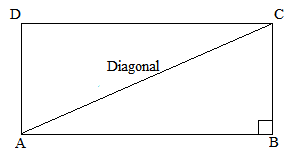(AC)2 = (AB)2   + (BC)2
(3x)2 = (x)2 + (y)2
9x2 = x2 + y2
8x2 = y2
Now, taking square roots of both sides, we get:
$2\sqrt{2}$ x = y
or,

Thus, the ratio of the larger side to the smaller side =  $2\sqrt{2}$  :   1

#### Question 7:

The ratio of the areas of two squares, one having its diagonal double than the other, is

(a) 1 : 2
(b) 2 : 3
(c) 3 : 1
(d) 4 : 1

#### Answer:

(d) 4 : 1

Let the two squares be ABCD and PQRS. Further, the diagonal of square PQRS is twice the diagonal of square ABCD.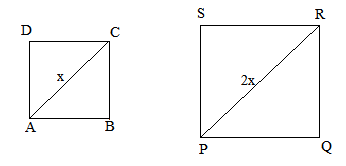PR  = 2 AC

Now, area of the square = $\frac{{\left(Diagonal\right)}^{2}}{2}$

Area of PQRS = $\frac{{\left(PR\right)}^{2}}{2}$

Similarly, area of ABCD = $\frac{{\left(AC\right)}^{2}}{2}$

According to the question:

If AC = x units, then, PR = 2x units

Therefore, = $\frac{{\left(PR\right)}^{2}×2}{2×{\left(AC\right)}^{2}}$ = $\frac{{\left(2x\right)}^{2}×2}{2×{\left(x\right)}^{2}}$ = $\frac{4}{1}$

Thus, the ratio of the areas of squares PQRS and ABCD = 4 : 1

#### Question 8:

If the ratio of areas of two squares is 225 : 256, then the ratio of their perimeters is

(a) 225 : 256
(b) 256 : 225
(c) 15 : 16
(d) 16 : 15

#### Answer:

(c) 15 : 16

Let the two squares be ABCD and PQRS.

Further, let the lengths of each side of ABCD and PQRS be x and y, respectively.

Therefore

= $\frac{{x}^{2}}{{y}^{2}}$

⇒ $\frac{{x}^{2}}{{y}^{2}}$= $\frac{225}{256}$

Taking square roots on both sides, we get:

$\frac{x}{y}$ = $\frac{15}{16}$

Now, the ratio of their perimeters:

= = $\frac{4x}{4y}$

⇒  = $\frac{x}{y}$

⇒ ​ = $\frac{15}{16}$

Thus, the ratio of their perimeters = 15 : 16

#### Question 9:

If the sides of a square are halved, the its area

(a) remains same
(b) becomes half
(c) becomes one fourth
(d) becomes double

#### Answer:

(c) becomes one fourth

Let the side of the square be x.

Then, area = (Side $×$ Side) = (x $×$ x) = x2

If the sides are halved, new side = $\frac{x}{2}$

Now, new area  = ${\left(\frac{x}{2}\right)}^{2}$$\frac{{x}^{2}}{4}$

It is clearly visible that the area has become one-fourth of its previous value.

#### Question 10:

A rectangular carpet has area 120 m2 and perimeter 46 metres. The length of its diagonal is

(a) 15 m
(b) 16 m
(c) 17 m
(d) 20 m

#### Answer:

(c) 17 m
Area of the rectangle = 120 m2
Perimeter = 46 m
Let the sides of the rectangle be l and b.
Therefore
Area = lb = 120 m2                 ...(1)
Perimeter = 2 (l + b) = 46
or,              ( l + b ) = $\frac{46}{2}$ = 23 m               ...(2)
Now, length of the diagonal of the rectangle =
So, we first find the value of (l2 + b2)
Using identity:
(l2 + b2 ) = (l + b)2 $-$ 2 (lb)             [From (1) and (2)]
Therefore
(l2 + b2) = (23)2 $-$ 2 (120)
= 529 $-$ 240 = 289
Thus, length of the diagonal of the rectangle = = $\sqrt{289}$ = 17 m

#### Question 11:

If the ratio between the length and the perimeter of a rectangular plot is 1 : 3, then the ratio between the length and breadth of the plot is

(a) 1 : 2
(b) 2 : 1
(c) 3 : 2
(d) 2 : 3

#### Answer:

(b) 2 : 1
It is given that = $\frac{1}{3}$
⇒  = $\frac{1}{3}$
After cross multiplying, we get:
3l = 2l + 2b
⇒ l = 2b
$\frac{l}{b}$= $\frac{2}{1}$
Thus, the ratio of the length and the breadth is 2 : 1.

#### Question 12:

If the length of the diagonal of a square is 20 cm, then its perimeter is

(a) $10\sqrt{2}\mathrm{cm}$
(b) 40 cm
(c) $40\sqrt{2}\mathrm{cm}$
(d) 200 cm

#### Answer:

(c) 40 $\sqrt{2}$cm

Length of the diagonal = 20 cm

Length of the side of a square = = $\frac{20}{\sqrt{2}}$ = $\frac{2×10}{\sqrt{2}}$
= $\frac{\sqrt{2}×\sqrt{2}×10}{\sqrt{2}}$ = 10$\sqrt{2}$ cm

Therefore, perimeter of the square = 4 $×$ Side = 4 $×$10$\sqrt{2}$ = 40$\sqrt{2}$ cm

#### Question 1:

Which of the following are closed curves? Which of them are simple?#### Answer:

Fig. (ii), (iii), (iv), (vi) and (vii) are closed curves, whereas fig. (ii), (iii), (iv) and (vi) are simple closed curves.

#### Question 2:

Define perimeter of a closed figure.

#### Answer:

The length of the boundary of a closed figure is known as its perimeter.

#### Question 3:

Find the perimeter of each of the following shapes:#### Answer:

Perimeter = Sum of lengths of all sides of a closed figure
(i)Perimeter = (4 + 2 + 1 + 5) cm = 12 cm

(ii)Perimeter = (23 + 35 + 40 + 35) cm = 133 cm

(iii)Perimeter = (15 + 15 + 15 + 15) cm = 60 cm

(iv)Perimeter = (3 + 3 + 3 + 3 + 3) cm = 15 cm

View NCERT Solutions for all chapters of Class 6